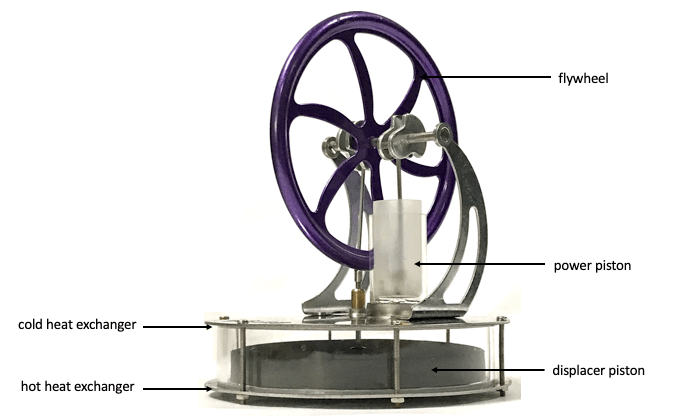## Uniform vertical circular motion

The following GeoGebra app simulates the force vectors on an object in uniform vertical circular motion.

A real world example of this would be the forces acting on a cabin in a ferris wheel.

<iframe scrolling="no" title="Vertical Uniform Circular Motion " src="https://www.geogebra.org/material/iframe/id/t5jstqsm/width/640/height/480/border/888888/sfsb/true/smb/false/stb/false/stbh/false/ai/false/asb/false/sri/true/rc/false/ld/false/sdz/false/ctl/false" width="640px" height="480px" style="border:0px;"> </iframe>

## Vertical Non-Uniform Circular Motion

This is a simulation that shows the vectors of forces acting on an object rolling in a vertical loop, assuming negligible friction.

To complete the loop, the initial velocity must be sufficiently high so that contact between the object and the track is maintained. When the contact force between the object and its looping track no longer exists, the object will drop from the loop.

The following code is for embedding in SLS.

<iframe scrolling="no" title="Vertical non-uniform circular motion" src="https://www.geogebra.org/material/iframe/id/ny3jhhsp/width/640/height/480/border/888888/sfsb/true/smb/false/stb/false/stbh/false/ai/false/asb/false/sri/true/rc/false/ld/false/sdz/false/ctl/false" width="640px" height="480px" style="border:0px;"> </iframe>

## Aircraft Turning in a Circle: a 3-D Visualisation with GeoGebra

This GeoGebra app is a 3-D visualisation tool of the force vectors acting on an aircraft turning with uniform circular motion in a horizontal plane.

I prepared this in advance as I will be lecturing on this JC1 topic next year.

## Hydrostatic Pressure and Upthrust

This app is used to demonstrate how a spherical object with a finite volume immersed in a fluid experiences an upthrust due to the differences in pressure around it.

Given that the centre of mass remains in the same position within the fluid, as the radius increases, the pressure due to the fluid above the object decreases while the pressure below increases. This is because hydrostatic pressure at a point is proportional to the height of the fluid above it.

It can also be used to show that when the volume becomes infinitesimal, the pressure acting in all directions is equal.

The following codes can be used to embed this into SLS.

<iframe scrolling="no" title="Hydrostatic Pressure and Upthrust" src="https://www.geogebra.org/material/iframe/id/xxeyzkqq/width/640/height/480/border/888888/sfsb/true/smb/false/stb/false/stbh/false/ai/false/asb/false/sri/false/rc/false/ld/false/sdz/false/ctl/false" width="640px" height="480px" style="border:0px;"> </iframe>

## Noise-cancelling AirPod Pro

The recently launched Apple AirPod Pro presents a wonderful opportunity to relate an A-level concept to a real-world example - how noise-cancelling earphones work.

Apple's website explained it in layman terms that seem to make sense. Let your students attempt to do a better job of explaining how destructive interference of waves is applied.

I probably won't spend SGD379 on it though.

## Why is Glass Transparent?

This video relates a phenomenon that we have taken for granted to the study of quantum physics (more specifically, photon absorption) and atomic structure.

## Phase Difference GeoGebra Apps

I created a series of GeoGebra apps for the JC topics of Waves and Superposition, mainly on the concept of Phase Difference. The sizes of these GeoGebra apps are optimised for embedding into SLS. When I have time, I will create detailed instructions on how to create such apps. Meanwhile, feel free to use them.

Instructions on how to embed the apps into SLS can be found at this staging environment of the SLS user guide.

Phase difference between two particles on a progressive wave. Move the particles along the wave to see the value.

Phase difference between two particles on a stationary wave. Move the particles along the wave to observe how their velocities are different or similar.

Observe velocity vectors of multiple particles on a progressive wave.

## Idealized Stirling Cycle

I created a new GeoGebra app based on an ideal Stirling Cycle (A. Romanelli Alternative thermodynamic cycle for the Stirling machine, American Journal of Physics 85, 926 (2017)) which includes two isothermal and two isochoric processes. The Stirling engine is a very good example to apply the First Law of Thermodynamics to, as the amount of gas is fixed so the macro-variables are only pressure, temperature and volume. Simplifying the cycle makes it even easier for first time learners to understand how the engine works.

For those who prefer to be impressed by an actual working model, it can be bought for less than S\$30 on Lazada. All you need for it to run is a little hot water or some ice. Here's a video of the one I bought:

The parts of the Stirling engine are labelled here:My simulation may not look identical to the engine shown but it does have the same power piston (to do work on the flywheel) and displacer piston (to shunt the air to and fro for more efficient heat exchange).

## Geogebra App on Maximum Power Theorem

This simulation demonstrates the power dissipated in a variable resistor given that the battery has an internal resistance (made variable in this app as well).

Since the power dissipated by the resistor is given by

$P=I^2R$

and the current is given by

$I=E(R+r)$,

$P= E^2\times\frac{R}{(R+r)^2}=\frac{E^2}{\frac{r^2}{R}+R+2r}$

This power will be a maximum if the expression for the denominator

$\frac{r^2}{R}+R+2r$

is a minimum.

Differentiating the expression with respect to R, we get

$\frac{d(r^2/R+R+2r)}{dR}=-\frac{r^2}{R^2}+1$

When the denominator is a minimum,

$-\frac{r^2}{R^2}+1=0$, so

r = R.